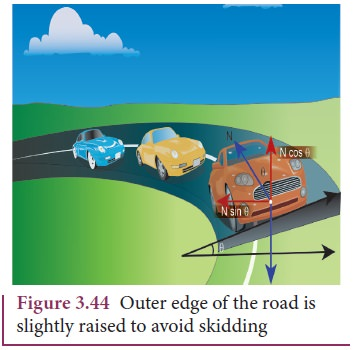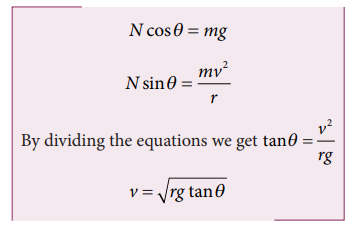Home | | Physics 11th std | Banking of Tracks

# Banking of Tracks

This introduces an inclination, and the angle is called banking angle.

Banking of Tracks

In a leveled circular road, skidding mainly depends on the coefficient of static friction μs The coefficient of static friction depends on the nature of the surface which has a maximum limiting value. To avoid this problem, usually the outer edge of the road is slightly raised compared to inner edge as shown in the Figure 3.44. This is called banking of roads or tracks. This introduces an inclination, and the angle is called banking angle.Let the surface of the road make angle θ with horizontal surface. Then the normal force makes the same angle θ with the vertical. When the car takes a turn, there are two forces acting on the car:

a.        Gravitational force mg (downwards)

b.        Normal force N (perpendicular to surface)

We can resolve the normal force into two components. N cosθ and N sinθ as shown in Figure 3.46. The component N cosθ balances the downward gravitational force ‘mg’ and component N sinθ will provide the necessary centripetal acceleration. By using Newton second lawThe banking angle θ and radius of curvature of the road or track determines the safe speed of the car at the turning. If the speed of car exceeds this safe speed, then it starts to skid outward but frictional force comes into effect and provides an additional centripetal force to prevent the outward skidding. At the same time, if the speed of the car is little lesser than safe speed, it starts to skid inward and frictional force comes into effect, which reduces centripetal force to prevent inward skidding. However if the speed of the vehicle is sufficiently greater than the correct speed, then frictional force cannot stop the car from skidding.

## Solved Example Problems for Banking of Tracks

### Example 3.25

Consider a circular road of radius 20 meter banked at an angle of 15 degree. With what speed a car has to move on the turn so that it will have safe turn?

### SolutionThe safe speed for the car on this road is 7.1 m s-1

Study Material, Lecturing Notes, Assignment, Reference, Wiki description explanation, brief detail
11th Physics : UNIT 3 : Laws of Motion : Banking of Tracks |